# Fórmula para calcular as probabilidades

• Nota de Estudos
• Rever Tópicos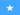Mohamed N. 0 0 how to got the answer ,still iam looking for it but.................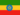Henock A. 0 0 done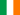Rachel N. 0 0 The formula for calculating probabilitiesThe formula for calculating probabilities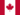Pushwi K. 0 0 Nice, but i wish solving a "Combination" has been explained.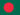Jahidul I. 0 0 The probability of an event tells that how likely the event will happen. Situations in which each outcome is equally likely, then we can find the probability using probability formula. Probability is a chance of prediction. If the probability that an event will occur is "x", then the probability that the event will not occur is "1 - x". If the probability that one event will occur is "a" and the independent probability that another event will occur is "b", then the probability that both events will occur is "ab". Probability of an event A can be written as: P(A) = Number offavorable outcomesTotal number of possible outcomes Probability is the measure of how likely an event is. And an event is one or more outcomes of an experiment. Probability formula is the ratio of number of favorable outcomes to the total number of possible outcomes. Probability Formula Probability of an Event = Number ofFavorable OutcomesTotal Number of Possible Outcomes Measures the likelihood of an event in the following way: - If P(A) > P(B) then event A is more likely to occur than event B. - If P(A) = P(B) then events A and B are equally likely to occur.Jahidul I. 0 0 what is Probability ?
• Text Version### Você recebeu uma nova notificação

Clique aqui para visualizar todos eles